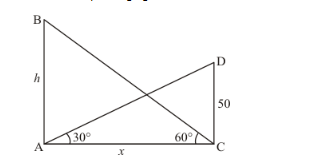# The angle of elevation of the top of a hill at the foot of a tower is 60° and the angle of elevation

Question:

The angle of elevation of the top of a hill at the foot of a tower is 60° and the angle of elevation of the top of the tower from the foot of the hill is 30°. If the tower is 50 m high, what is the height of the hill?

Solution:

Letbe the height of hill. Andbe the tower of heightm. Angle of elevation of the top of hill from the foot of tower is 60° and angle of elevation of top of tower from foot of hill is 30°. Letand,Here we have to find height of hill.

The corresponding figure is as followsSo we use trigonometric ratios.

In,

$\Rightarrow \quad \tan 30^{\circ}=\frac{C D}{A C}$

$\Rightarrow \quad \frac{1}{\sqrt{3}}=\frac{50}{x}$

$\Rightarrow \quad x=50 \sqrt{3}$

Again in $\triangle A B C$

$\Rightarrow \quad \tan 60^{\circ}=\frac{A B}{A C}$

$\Rightarrow \quad \sqrt{3}=\frac{h}{x}$

$\Rightarrow \quad h=x \sqrt{3}$

$\Rightarrow \quad h=150$

Hence the height of hill is $150 \mathrm{~m}$.